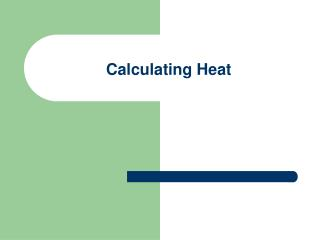DownloadDownload PresentationCalculating Heat

# Calculating Heat

Download Presentation## Calculating Heat

- - - - - - - - - - - - - - - - - - - - - - - - - - - E N D - - - - - - - - - - - - - - - - - - - - - - - - - - -
##### Presentation Transcript

1. Calculating Heat

2. Specific Heat • Amount of heat energy needed to raise the temp of 1 ml of a substance 1°C • For water the specific heat is 4.19 J/g °C, but it is differenct for different substances • It takes 4.19 J to raise the temp of 1 g of water 1 °C • Every substance has a different specific heat

3. Table of Specific Heats

4. Calculating Heat When Substance in One Phase • Heat released or absorbed can be calculated by multiplying three factors • Heat = specific heat x mass x temp change q = c x m x Δt

5. Practice • The specific heat of water is 4.19 J/ g C. How much heat is needed to warm 350g of water from 25 C to 75 C? • If 178 J of heat is needed to increase the temperature of a 20g piece of copper metal from 25 C to 48 C, what is the specific heat of copper?

6. Heat and Phase Change (Evaporation) • Heat of vaporization (Hvao)energy required to change one gram of a substance from liquid to gas. • For water 2260J/g • Q = Hvao m

7. Practice • The heat of vaporization of water is 2260J/g. How much heat must be supplied to evaporate 50g of water? • How much heat is required to evaporate 150.g of a substance at its boiling point if it has a Hvap= 987 J/g?

8. Energy and Phase Change • Heat of fusion (Hfus) energy required to change one gram of a substance from solid to liquid. • For water 340 J/g • Q = Hfus m

9. Examples • The heat of fusion of ice at 0°C is 340J/g. How much heat is needed to change 75g of ice at 0°C to a liquid at the same temperature? • The heat of fusion of water at 0°C is 340J/g. How much heat is released when 250g of water changes to ice at 0°C?

10. Practice • How much heat is needed to change 100g of water at 50C to steam at 120C? The specific heat of water is 4.19 J/g C, the specific heat of steam is 1.7 J/g C, and the heat of vaporization of water is 2260 J/g. • How much heat must be released as 50g of water at 25C to ice at -10C? The specific heat of water is 4.19 J/g C, the specific heat of ice is 2.1 J/g C, and the heat of fusion fro water is 340 J/g.